•#### R for Data Science#### Overview of this book

R for Data ScienceCreditswww.PacktPub.comPrefaceFree Chapter
Data Mining PatternsData Mining SequencesText MiningData Analysis – Regression AnalysisData Analysis – CorrelationData Analysis – ClusteringData Visualization – R GraphicsData Visualization – PlottingData Visualization – 3DMachine Learning in ActionPredicting Events with Machine LearningSupervised and Unsupervised LearningIndex## Anomaly detection

We can use R programming to detect anomalies in a dataset. Anomaly detection can be used in a number of different areas, such as intrusion detection, fraud detection, system health, and so on. In R programming, these are called outliers. R programming allows the detection of outliers in a number of ways, as listed here:

• Statistical tests

• Depth-based approaches

• Deviation-based approaches

• Distance-based approaches

• Density-based approaches

• High-dimensional approaches

### Show outliers

R programming has a function to display outliers: `identify` (in `boxplot`).

The `boxplot` function produces a box-and-whisker plot (see following graph). The `boxplot` function has a number of graphics options. For this example, we do not need to set any.

The `identify` function is a convenient method for marking points in a scatter plot. In R programming, box plot is a type of scatter plot.

#### Example

In this example, we need to generate a 100 random numbers and then plot the points in boxes.

Then, we mark the first outlier with it's identifier as follows:

```> y <- rnorm(100)
> boxplot(y)
> identify(rep(1, length(y)), y, labels = seq_along(y))```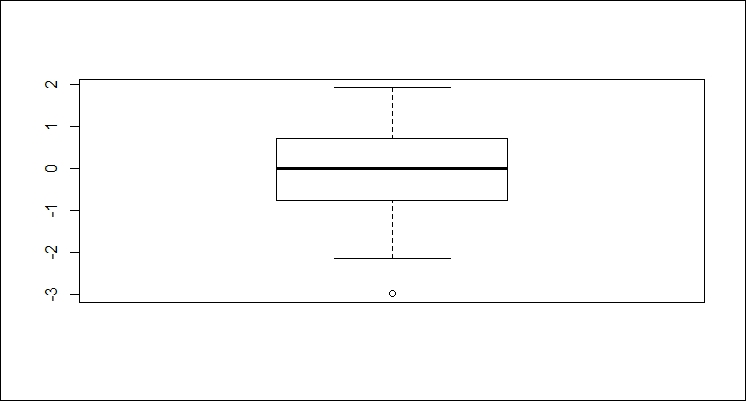### Note

Notice the 0 next to the outlier in the graph.

#### Example

The `boxplot` function automatically computes the outliers for a set as well.

First, we will generate a 100 random numbers as follows (note that this data is randomly generated, so your results may not be the same):

`> x <- rnorm(100)`

We can have a look at the summary information on the set using the following code:

```> summary(x)
Min.  1st Qu.   Median     Mean  3rd Qu.     Max.
-2.12000 -0.74790 -0.20060 -0.01711  0.49930  2.43200```

Now, we can display the outliers using the following code:

```> boxplot.stats(x)\$out
 2.420850 2.432033```

The following code will graph the set and highlight the outliers:

`> boxplot(x)`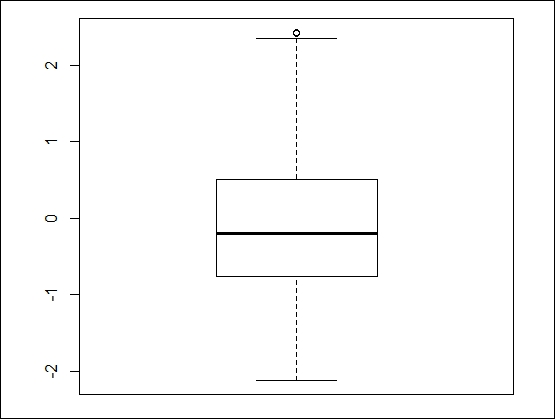### Note

Notice the 0 next to the outlier in the graph.

We can generate a box plot of more familiar data showing the same issue with outliers using the built-in data for cars, as follows:

`boxplot(mpg~cyl,data=mtcars, xlab="Cylinders", ylab="MPG")`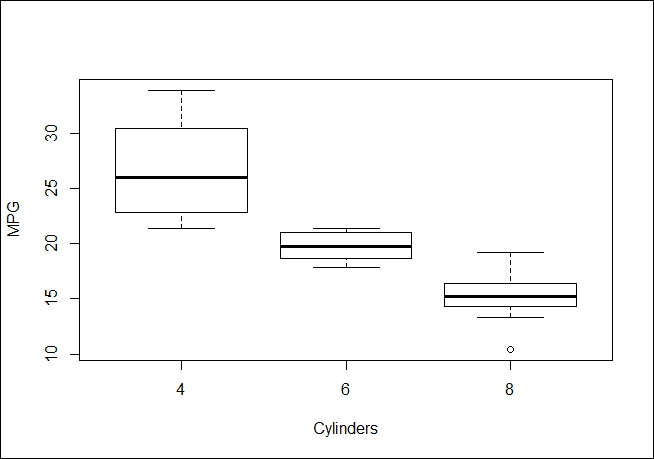#### Another anomaly detection example

We can also use box plot's outlier detection when we have two dimensions. Note that we are forcing the issue by using a union of the outliers in `x` and `y` rather than an intersection. The point of the example is to display such points. The code is as follows:

```> x <- rnorm(1000)
> y <- rnorm(1000)
> f <- data.frame(x,y)
> a <- boxplot.stats(x)\$out
> b <- boxplot.stats(y)\$out
> list <- union(a,b)
> plot(f)
> px <- f[f\$x %in% a,]
> py <- f[f\$y %in% b,]
> p <- rbind(px,py)
> par(new=TRUE)
> plot(p\$x, p\$y,cex=2,col=2)```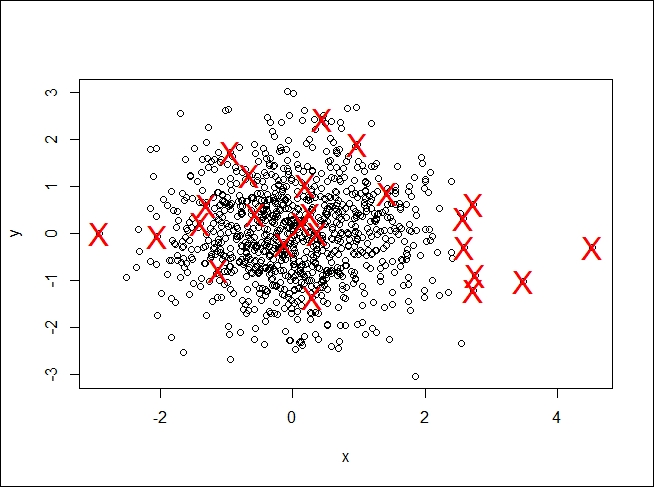While R did what we asked, the plot does not look right. We completely fabricated the data; in a real use case, you would need to use your domain expertise to determine whether these outliers were correct or not.

### Calculating anomalies

Given the variety of what constitutes an anomaly, R programming has a mechanism that gives you complete control over it: write your own function that can be used to make a decision.

#### Usage

We can use the `name` function to create our own anomaly as shown here:

```name <- function(parameters,…) {
# determine what constitutes an anomaly
return(df)
}```

Here, the parameters are the values we need to use in the function. I am assuming we return a data frame from the function. The function could do anything.

#### Example 1

We will be using the `iris` data in this example, as shown here:

`> data <- read.csv("http://archive.ics.uci.edu/ml/machine-learning-databases/iris/iris.data")`

If we decide an anomaly is present when sepal is under 4.5 or over 7.5, we could use a function as shown here:

```> outliers <- function(data, low, high) {
>  outs <- subset(data, data\$X5.1 < low | data\$X5.1 > high)
>  return(outs)
>}```

Then, we will get the following output:

```> outliers(data, 4.5, 7.5)
X5.1 X3.5 X1.4 X0.2    Iris.setosa
8    4.4  2.9  1.4  0.2    Iris-setosa
13   4.3  3.0  1.1  0.1    Iris-setosa
38   4.4  3.0  1.3  0.2    Iris-setosa
42   4.4  3.2  1.3  0.2    Iris-setosa
105  7.6  3.0  6.6  2.1 Iris-virginica
117  7.7  3.8  6.7  2.2 Iris-virginica
118  7.7  2.6  6.9  2.3 Iris-virginica
122  7.7  2.8  6.7  2.0 Iris-virginica
131  7.9  3.8  6.4  2.0 Iris-virginica
135  7.7  3.0  6.1  2.3 Iris-virginica```

This gives us the flexibility of making slight adjustments to our criteria by passing different parameter values to the function in order to achieve the desired results.

#### Example 2

Another popular package is `DMwR`. It contains the `lofactor` function that can also be used to locate outliers. The `DMwR` package can be installed using the following command:

```> install.packages("DMwR")
> library(DMwR)```

We need to remove the species column from the data, as it is categorical against it data. This can be done by using the following command:

`> nospecies <- data[,1:4]`

Now, we determine the outliers in the frame:

`> scores <- lofactor(nospecies, k=3)`

Next, we take a look at their distribution:

`> plot(density(scores))`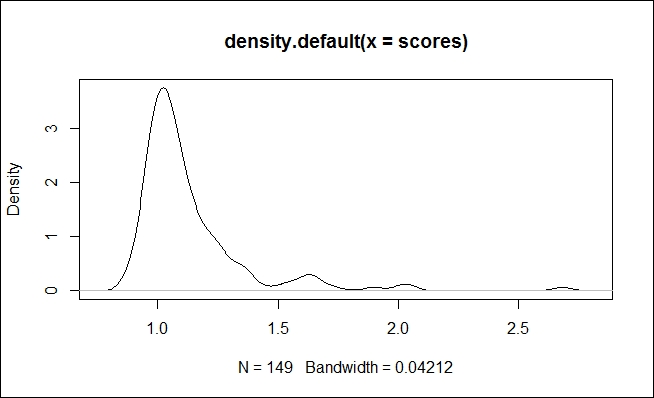One point of interest is if there is some close equality amongst several of the outliers (that is, density of about 4).